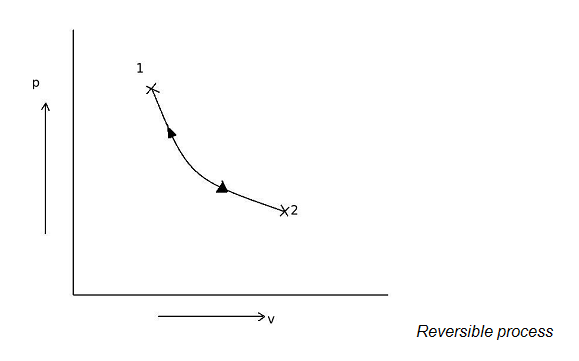# In which process, every state passes by the system is an equilibrium state ?

## In which process, every state passes by the system is an equilibrium state ?

a. quasi-static process
b. reversible process
c. both a. and b.
d. none of the above

Correct Answer : c. both a. and b.

Explanation :

A reversible process is the process in which, after completing the process, both the system and surroundings can be restored to their initial state, without producing any change is the rest of the universe. equivalence of kelvin planck and clausius statement.Let us consider a system is at state 1 and it is taken to the state 1 quasi-statically by following a path 1-2. Again the system is taken reversibly to its initial state 1 from state 2 again quasi-statically by following the same path 2-1. Then this process is called reversible process. A reversible process is carried out infinitely slowly, so that every state passed through by the system is an equilibrium state. Thus reversible process coincides with a quasi-static process.